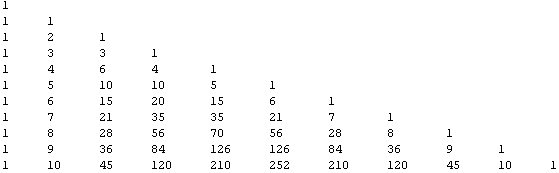# The Programmatic Side of Mathematica IV: Functions Associated with Pascal’s Triangle

The nth triangular number can be written as a function:

`triangular[n_] := Sum[i, {i, 1, n}]`

What about a series of such numbers? You may sequentialize triangular[n] and make it return an array:

`Table[triangular[n], {n, 1, 100}]`

But it’s better to give an arbitrary length array:

`triarr[n_] := Table[triangular[m], {m, 1, n}]`

so that we can do things like obtain the first 25 triangular numbers:

```triarr

{1,3,6,10,15,21,28,36,45,55,66,78,91,105,120,136,153,171,190,210,231,253,276,\
300,325}```

And while we’re at it, we can declare another function for tetrahedral numbers:

`tetrahedral[m_] := Apply[Plus, triarr[m]]`

which gives the nth tetrahedral number. And this gets us into the world of something called “n-Simplex” numbers. All of the n-Simplex numbers can be illustrated on Pascal’s triangle. Pascal’s triangle itself can be generated by masssaging a Mathematica function, although the shape of the triangle is not the familiar isosceles one:

```pascal[p_] := TableForm[Table[Binomial[n, k], {n, 0, p}, {k, 0, n}]]

pascal```But it is still kind of OK, since now we have the triangular numbers arranged in a column instead of a diagonal. In this case, the third column from the left. The tetrahedral numbers are in the fourth column, the 4-Simplex numbers (alternatively called the pentatopic or pentachron numbers) in the fifth column, the 5-Simplex numbers in the sixth column and so on. Like the tetrahedral numbers which arise from the sums of the first n triangular numbers, the nth k-simplex numbers generally are the sums of the first n (k-1) simplex numbers.

I imagine that the reason the numbering of “k-simplex” numbers are one behind their popular names (pentatopic seems to suggest “5” in the name, yet it is a 4-simplex set of numbers) is because the counting starts from 0 in column 1. While I haven’t read much on this, I am guessing that the “0-Simplex” numbers must be a repeating set of 1’s going forever, like we have in the first column, which I ought to be calling “column zero,” since it matches all Pascal’s triangle members with their combinations formulae. The “1-Simplex’ numbers are just the set of natural numbers in sequence from 1 to infinity. The “2-Simplex” numbers are the triangular numbers; and the “3-Simplex” numbers are the tetrahedral numbers. This brings us back to the pentatopic “4-Simplex” numbers. It seems fitting, anyway.

The Simplex system is supposed to also relate to geometry such that 0-Simplex means 0 dimensions or a point. 1-Simplex can be represented by a line, or points joined together in one dimension. 2-Simplex, our triangular numbers, fit in two dimensions; 3-simplex, the tetrahedral numbers, fit in 3-dimensions. The triangle is a shape that has the fewest sides that can fit in 2 dimensions, while the tetrahedron is a shape that has the fewest sides that can fit in 3 dimensions. After that, we are discussing shapes with “hyperfaces” in hyperdimensional space. The rest of this discussion can be found on Wikipedia.

You might also recall that each row in Pascal’s triangle represents the coefficients of the binomial expansion of (a + b)n.

```binexpand[z_] := Expand[(a + b)^z]

binexpand```

whose output becomes:$a^7 + 7a^6b + 21a^5b^2 + 35a^4b^3 + 35a^3b^4 + 21a^2b^5 + 7ab^6 + b^7$, the binomial expansion of$(a + b)^7$.